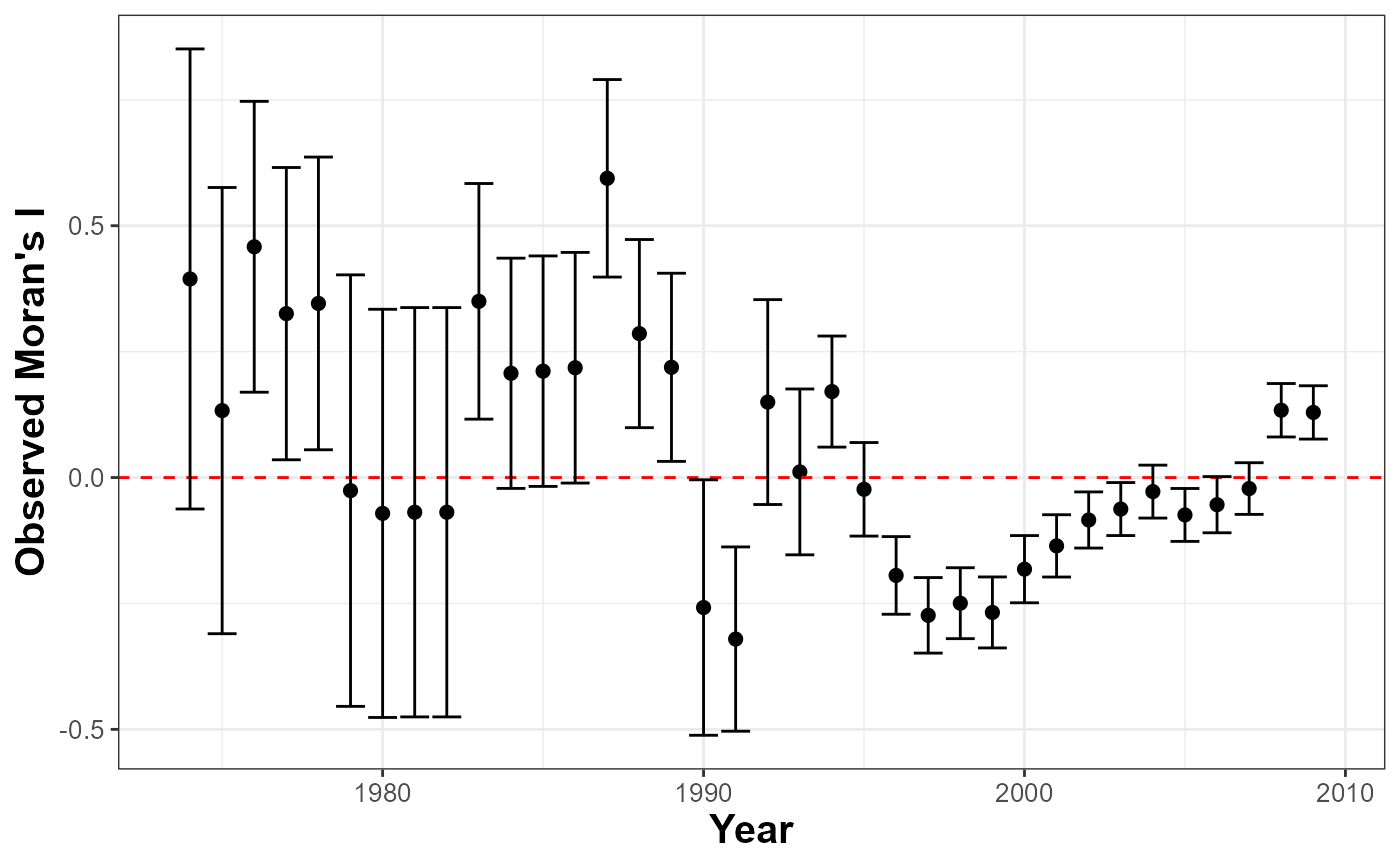Implements Global Moran I test to evaluate spatial autocorrelation in a units' risk propensity in the data. Positive values indicate spatial clustering of similar values.

plot_Moran.I(
data,
var_duration = character(),
var_id = character(),
var_time = character(),
n = 1,
t = 1.645,
threshold = 800L
)

## Arguments

data data. variable that measures duration until censoring or failure. ID's unique identifier. variable that measures time. number of observation per id. value of the confidence interval. distance in kilometers. By default is 800.

A ggplot object

## Examples


library(BayesSPsurv)
dataw <- spduration::add_duration(data = BayesSPsurv::Walter_2015_JCR,
y = "renewed_war",
unitID = "ccode",
tID = "year",
freq = "year",
ongoing = FALSE)#> Warning: Converting to 'Date' class with yyyy-06-30

plot_Moran.I(data = dataw ,
var_duration = "duration",
var_id = "ccode",
var_time = "year",
n = 12)